# $pandas.DataFrame()$的$loc$与$iloc$用法

$pandas.DataFrame()$实际上是一个二维矩阵，$loc$$iloc$方法都是用于从$pandas.DataFrame()$中选取一些行，一些列，或者具体的某个位置的值，也可以间接的来改变$DataFrame$里面的满足条件的行，列，或具体位置的值，$loc$$iloc$的区别就在于$loc$$Selection\ by\ Label$ (即通过标签索引)，而$iloc$$Selection\ by\ Position$ (即通过$DataFrame$的下标索引，$DataFrame$行列下标都是$0,1,2...$)

• ## 函数原型

###### $df.loc[row\_indexer,column\_indexer]$ ($iloc$也一样)

其中$row\_indexer$$column\_indexer$分别指行和列的索引方法，下文要讲的就是各种各样的索引方法，每种方法即可作为$row\_indexer$也可以作为$column\_indexer$，所以可以自由组合（但是只能$loc$$loc$的方法组合，$iloc$$iloc$的方法组合，不可交叉）,在不指定$column\_indexer$的情况下默认全部选，但是如果不指定$row\_indexer$的话需要这样写$DataFrame.loc[:,column\_indexer]$

• ## $pandas.DataFrame.loc$

$loc$方法通过标签或者bool数组选取一些行和列，首先构造一个DataFrame，作为全文解析用的例子：

import pandas as pd
import numpy as np  #numpy后面要用到

df=pd.DataFrame([[1,2,3,4],[5,6,7,8],[9,10,11,12],[13,14,15,16]]
,columns=['Chinease','Math','English','Politics']
,index=['Bob','Cindy','Alice','QWQ'])
print(df)


也就是这样的一个DataFrame：

Chinease Math English Politics
Bob 1 2 3 4
Cindy 5 6 7 8
Alice 9 10 11 12
QWQ 13 14 15 16

$loc$的几种使用方法如下所述：

• 通过单个标签选取一行或者一列，如 ‘Alice’
如选取Alice这一行：
print(df.loc['Alice'])   #返回值类型为Series

输出如图：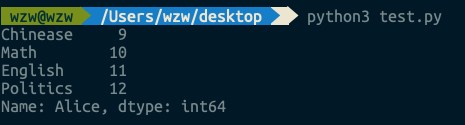当然也可以选取一列：
print(df.loc[:,'Math'])

输出如图：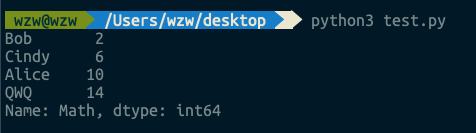对应的修改某一行或者某一列的所有值的操作为：
df.loc['Bob']=10 #修改Bob这一行的所有值为10
df.loc[:,'English']=0 #修改English这一列的所有值为0
df.loc['Bob','English']=0 #修改Bob同学的English成绩为0

剩下的方法对应的改值方法类似，故下面不再赘述
• 通过标签的一个列表或者一个array来选取一些行
如选取Bob和Alice这两行
print(df.loc[['Bob','Alice']])  #返回值类型为DataFrame

运行结果：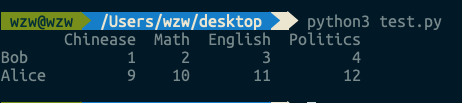也可以通过使用numpy.array来索引：
print(df.loc[np.array(['Bob','Alice'])])

运行结果与上图一致
• 通过标签的一个切片$(slice)$来选取一些连续的行，这里需要注意两点，第一这里是标签的切片，比如 ‘Bob’:‘Alice’ 这样，第二就是这里的切片有一点不同于python里的普通切片：这里的切片包含上界
比如选取从Bob到Alice之间的所有行：
print(df.loc['Bob':'Alice']) #返回值类型为DataFrame

运行结果如图：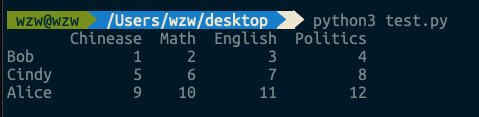• 通过一个与轴等长的$bool$数组选取一些行，这里的数组实际上可以是$list$或者$numpy.array$或者是值类型为$bool$$Series$
比如选取 Bob, Alice, QWQ 这三行:
print(df.loc[[False,False,True,True]])  #list
print(df.loc[np.array([False,False,True,True])]) #numpy.array
print(df.loc[df['English']>8]) #语句df['English']>8返回的就是一个只为布尔类型的Series

三种写法效果相同：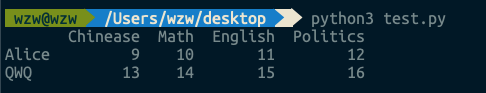• 上述四种方法可以两两组合来同时索引行和列
例如：
print(df.loc[df['English']>6,[True,False,True,True]]) #取English大于6的行与第0，2，3列的交集

输出如下所示：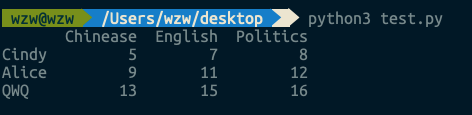• ## $pandas.DataFrame.iloc$

$iloc$方法通过下标（即整数）访问一些行或者列，只是把$loc$方法中采用的标签索引改成下表访问了而已，方法与上述方法相同，故这里只给出一些例子，读者需要用的话可以自行运行测试

• 通过一个整数下标访问：
df.iloc #返回df的第0行，返回值类型为Series

• 通过一个包含整数的$list$访问：
df.iloc[[0,2,3]] #返回df的第0，2，3行，返回值类型为DataFrame

• 通过一个切片访问，注意这里的切片和不同的切片一样，即不包含上界
df.iloc[1:3] #返回df的第1，2行，返回值类型为DataFrame

• 通过一个与轴等长的布尔数组访问
df.iloc[[True,False,True,True]] #返回df中第0，2，3行，返回值类型为DataFrame

• 上述四种方式可以两两组合来同时索引行和列
例如：
print(df.iloc[0:3,[True,False,True,True]]) #选取第0，1，2行与0，2，3列的交集，返回值类型为DataFrame

• ## 参考

©️2019 CSDN 皮肤主题: 技术黑板 设计师: CSDN官方博客